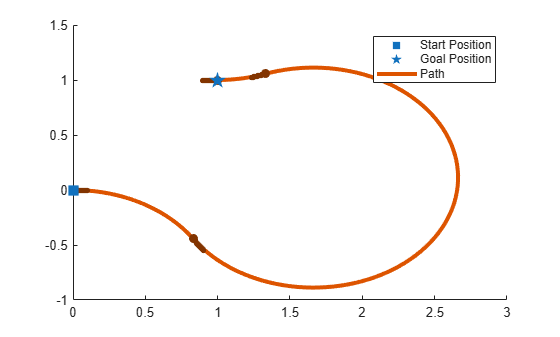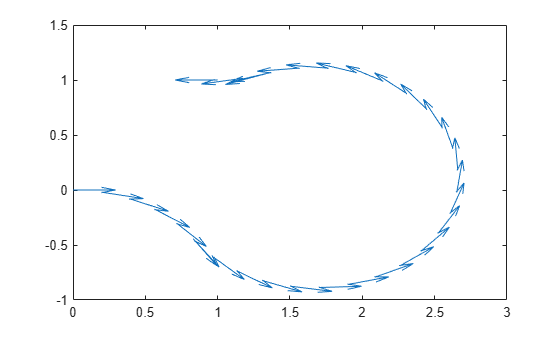# interpolate

Interpolate poses along path segment

## Syntax

``poses = interpolate(pathSeg)``
``poses = interpolate(pathSeg,lengths)``
``[poses,directions] = interpolate(___)``

## Description

````poses = interpolate(pathSeg)` interpolates along the path segment at the transitions between motion types.```

example

````poses = interpolate(pathSeg,lengths)` interpolates along the path segment at the specified lengths along the path. Transitions between motion types are always included.```
````[poses,directions] = interpolate(___)` also returns the direction of motion along the path for each section as a vector of 1s (forward) and –1s (reverse) using the previous inputs.```

## Examples

collapse all

Create a `dubinsConnection` object.

`dubConnObj = dubinsConnection;`

Define start and goal poses as `[x y theta]` vectors.

```startPose = [0 0 0]; goalPose = [1 1 pi];```

Calculate a valid path segment to connect the poses.

`[pathSegObj,pathCosts] = connect(dubConnObj,startPose,goalPose);`

Show the generated path.

`show(pathSegObj{1})`Interpolate poses along the path. Get a pose every 0.2 meters, including the transitions between turns.

```length = pathSegObj{1}.Length; poses = interpolate(pathSegObj{1},0:0.2:length)```
```poses = 32×3 0 0 0 0.1987 -0.0199 6.0832 0.3894 -0.0789 5.8832 0.5646 -0.1747 5.6832 0.7174 -0.3033 5.4832 0.8309 -0.4436 5.3024 0.8418 -0.4595 5.3216 0.9718 -0.6110 5.5216 1.1293 -0.7337 5.7216 1.3081 -0.8226 5.9216 ⋮ ```

Use the `quiver` function to plot these poses.

`quiver(poses(:,1),poses(:,2),cos(poses(:,3)),sin(poses(:,3)),0.5)`## Input Arguments

collapse all

Lengths along path to interpolate at, specified as a positive numeric vector. For example, specify `[0:0.1:pathSegObj{1}.Length]` to interpolate at every 0.1 meters along the path. Transitions between motion types are always included.

## Output Arguments

collapse all

Interpolated poses along the path segment, specified as an [x, y, Θ] matrix. Each row of the matrix corresponds to a different interpolated pose along the path.

x and y are in meters. Θ is in radians.

Directions of motion for each segment of the interpolated path, specified as a vector of 1s (forward) and –1s (reverse).

## Version History

Introduced in R2019b